﻿ Project Maths | Learn to use GeoGebra
Working together to improve teaching & learning Leagan Gaeilge
For students ›› Learn to use GeoGebra

## Why do maths students use GeoGebra?

GeoGebra is a simple but powerful tool which can be used by students to understand maths concepts, to help solve problems and to check solutions. GeoGebra can be used for statistics, for probability, for geometry, for trigonometry, for algebra and for functions. You can learn the basics of GeoGebra really quickly and in no time you’ll see your maths in a whole new way.

## GeoGebra tutorials

To help you get started on using GeoGebra here are some short videos which explain the basics of GeoGebra.

Download GeoGebra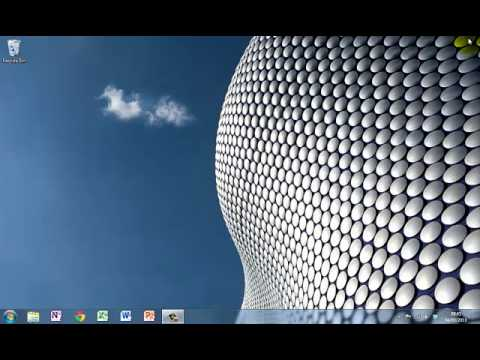Set up GeoGebra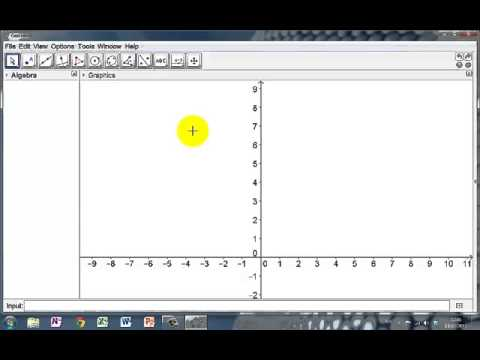This tutorial shows you how to change some basic settings on GeoGebra to make it eas...

Move the graphics view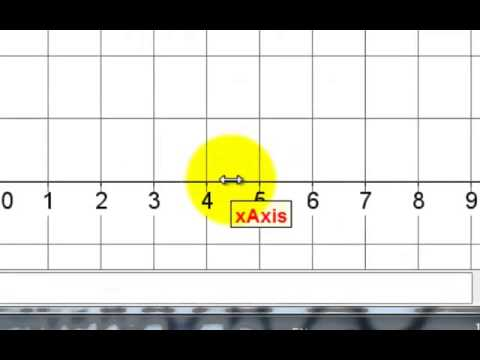Moving and scaling the graphics view is one of the most useful things to know In Geo...

Inserting a picture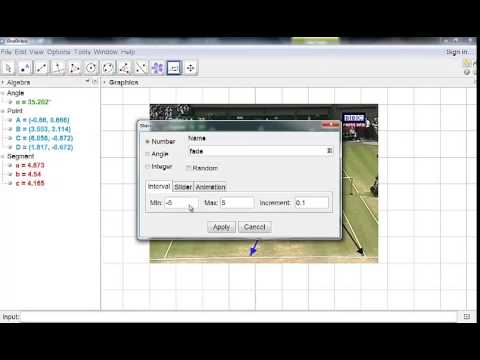When linking maths to real-life it ccan be useful to insert a picture into GeoGebra....

Introduction to geometry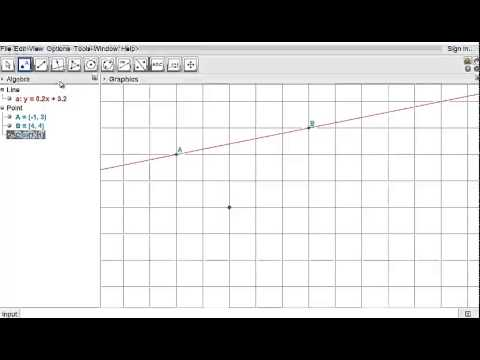Knowledge of a few basic tools makes it easy to learn Geometry using Geogebra. This ...

Bisect a line segment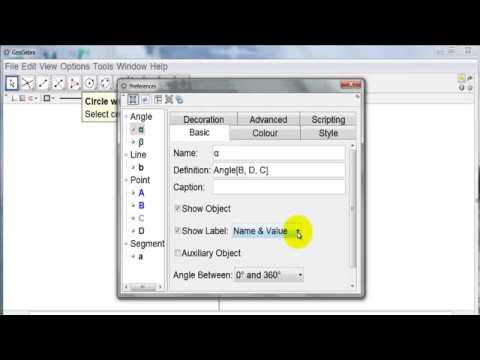Want to construct the perpendicular bisector of a line segment? This tutorial will s...

The equation of a circleUnderstanding the equation of a circle is so much easier when you can see the circle...

Introduction to statistics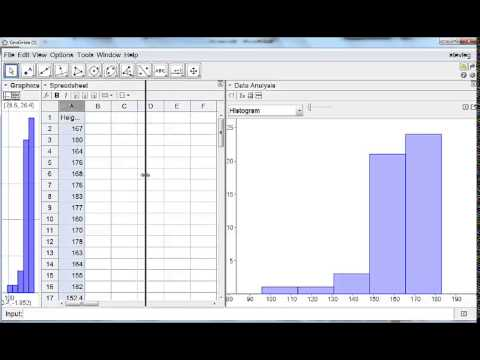Use GeoGebra with Census@SChool data to create charts, calculate means, standard dev...

## What is GeoGebra?

GeoGebra is a free dynamic mathematics software package used by teachers of mathematics in classrooms across the world.  It joins geometry, algebra, tables, graphing, statistics and calculus in one easy-to-use package. It has won several educational software awards across the globe.